Most Affordable JEE | NEET | 8,9,10 Preparation by Kota's Top IITian Doctor Faculties

# NCERT Solutions for Class 9 Maths chapter 6 Exercise 6.1 - Lines and Angles

Class 9Hey, are you a class 9 Student and Looking for Ways to Download NCERT Solutions for Class 9 Maths chapter 6 Exercise 6.1? If Yes then you are at the right place.

Here we have listed Class 9 maths chapter 6 exercise 6.1 solutions in PDF that is prepared by Kota’s top IITian’s Faculties by keeping Simplicity in mind.

If you want to score high in your class 9 Maths Exam then it is very important for you to have a good knowledge of all the important topics, so to learn and practice those topics you can use eSaral NCERT Solutions.

So, without wasting more time Let’s start.

### Download The PDF of NCERT Solutions for Class 9 Maths chapter 6 Exercise 6.1 "Lines and Angles"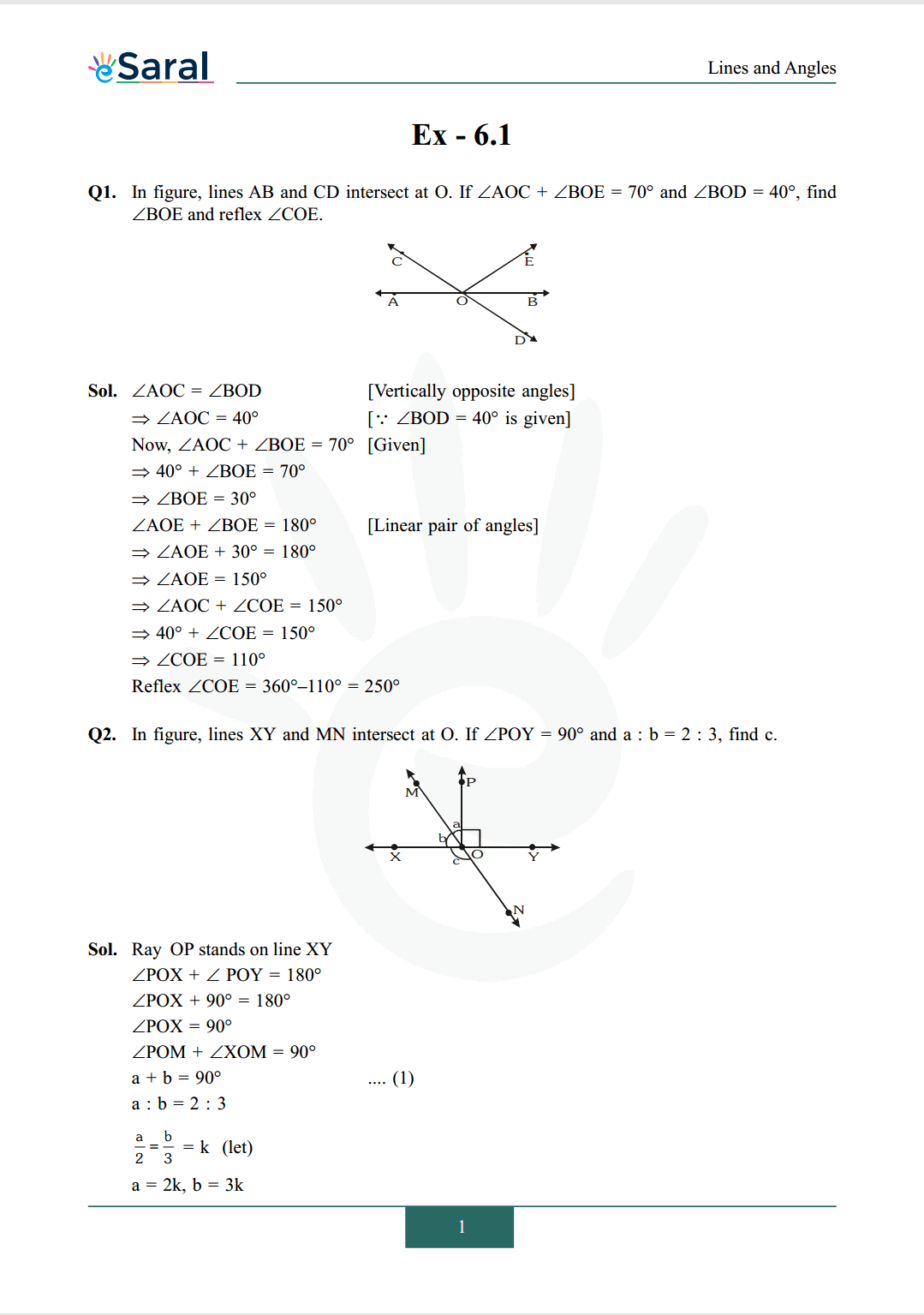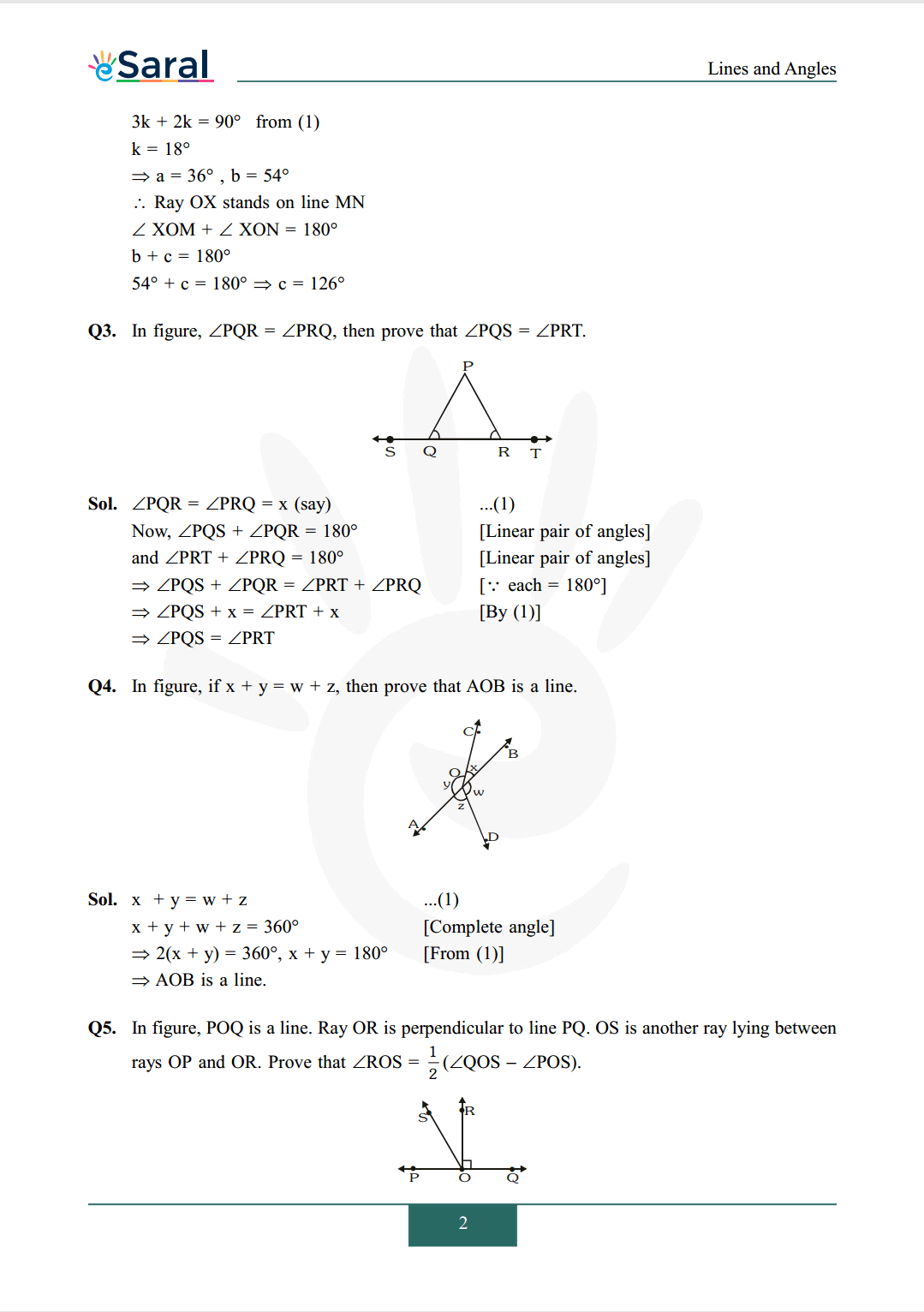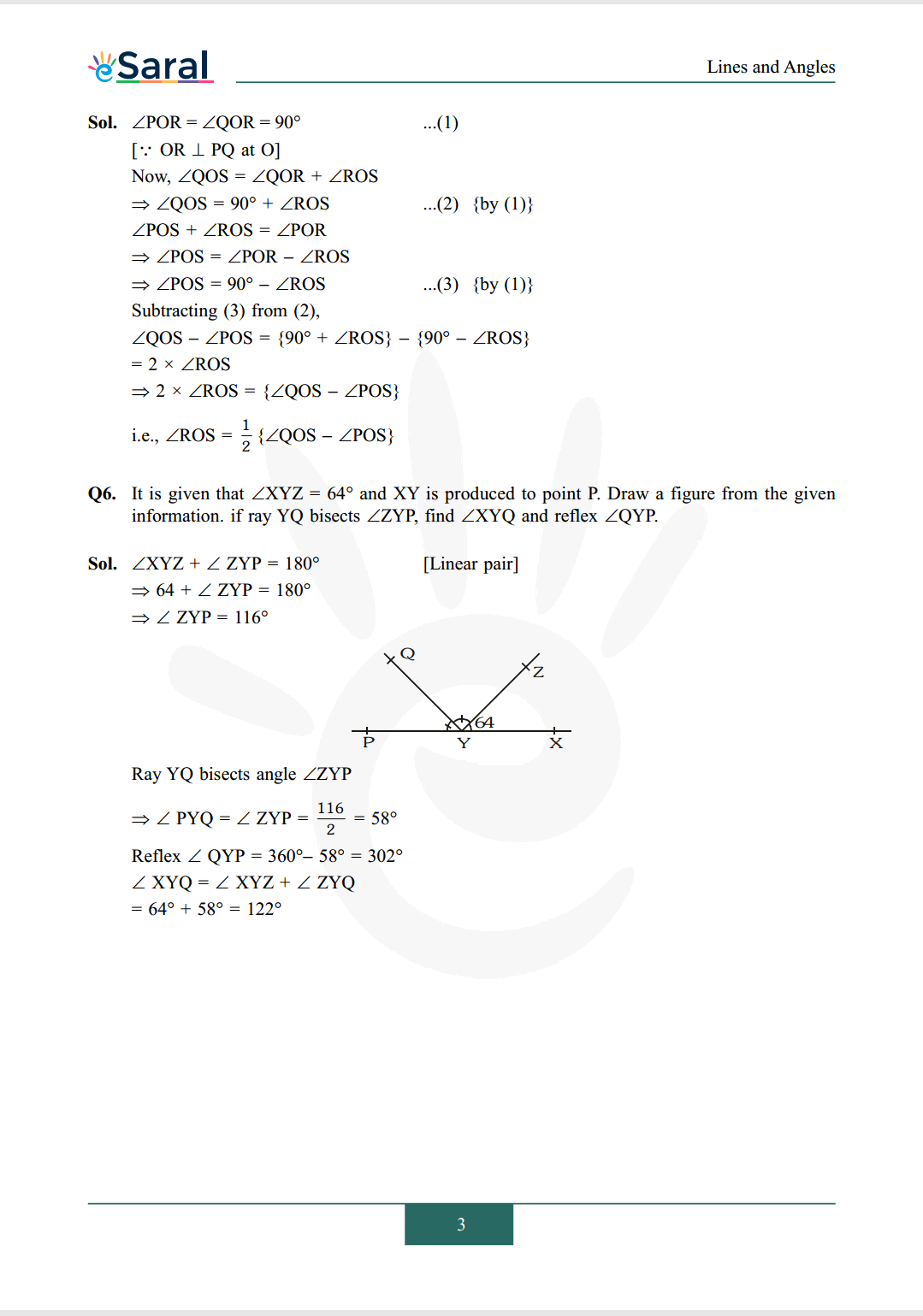#### All Questions of Chapter 6 Exercise 6.1

Once you complete the chapter 6 then you can revise Ex. 6.1 by solving following questions

Q1. In figure, lines $\mathrm{AB}$ and $\mathrm{CD}$ intersect at $\mathrm{O} .$ If $\angle \mathrm{AOC}+\angle \mathrm{BOE}=70^{\circ}$ and $\angle \mathrm{BOD}=40^{\circ}$, find $\angle \mathrm{BOE}$ and reflex $\angle \mathrm{COE}$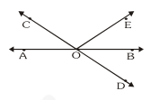Q2. In figure, lines $\mathrm{XY}$ and $\mathrm{MN}$ intersect at $\mathrm{O}$. If $\angle \mathrm{POY}=90^{\circ}$ and $\mathrm{a}: \mathrm{b}=2: 3$, find $\mathrm{c}$.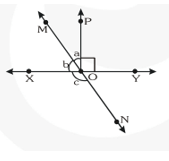Q3. In figure, $\angle \mathrm{PQR}=\angle \mathrm{PRQ}$, then prove that $\angle \mathrm{PQS}=\angle \mathrm{PRT}$.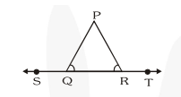Q4. In figure, if $x+y=w+z$, then prove that $A O B$ is a line.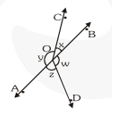Q5. In figure, $\mathrm{POQ}$ is a line. Ray OR is perpendicular to line PQ. OS is another ray lying between rays OP and OR. Prove that $\angle \operatorname{ROS}=\frac{1}{2}(\angle$ QOS $-\angle P O S)$.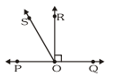Q6. It is given that $\angle \mathrm{XYZ}=64^{\circ}$ and $\mathrm{XY}$ is produced to point $\mathrm{P}$. Draw a figure from the given information. if ray YQ bisects $\angle Z Y P$, find $\angle X Y Q$ and reflex $\angle Q Y P$.

Class 9 NCERT Maths Book Chapterwise PDF

Class 9 NCERT Maths Exemplar Chapterwise PDF

Complete Solutions for Class 9 chapter 6